## Example Questions

### Example Question #29 : Trigonometry

Which of the following equations represents a tangent function with a period that is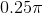radians?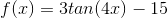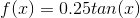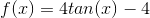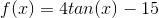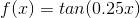Explanation:

The standard period of a tangent function isradians. In other words, it completes its entire cycle of values in that many radians. To alter the period of the function, you need to alter the value of the parameter of the trigonometric function. You multiply the parameter by the number of periods that would complete inradians. With a period of, you are quadrupling your method. Therefore, you will have a function of the form: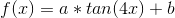Sinceanddo not alter the period, these can be anything.

Therefore, among your options,is correct.

### Example Question #1 : How To Find The Period Of The Tangent

Which of the following represents a tangent function that has a period half that of one with a period of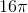?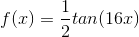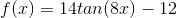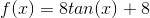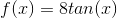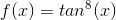Explanation:

The standard period of a tangent function isradians. In other words, it completes its entire cycle of values in that many radians. To alter the period of the function, you need to alter the value of the parameter of the trigonometric function. You multiply the parameter by the number of periods that would complete inradians. With a period of, you are multiplying your parameter by. Now, half of this would be a period of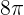. Thus, you will have a function of the form: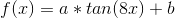Sinceanddo not alter the period, these can be anything.

Therefore, among your options,is correct.

### Example Question #31 : Trigonometry

What is the period of the following tangent function?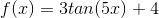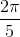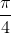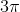Explanation:

The period of the tangent function defined in its standard form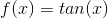has a period of. When you multiply the argument of the trigonometric function by a constant, you shorten its period of repetition. (Think of it like this: You pass through more iterations for each valuethat you use.) If you have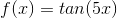, this has one fifth of the period of the standard tangent function.  In the equation given, none of the other details matter regarding the period. They alter other aspects of the equation (its "width," its location, etc.). The period is altered only by the parameter. Thus, the period of this function isof, or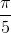.

### Example Question #32 : Trigonometry

What is the period of the following trigonometric equation: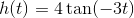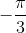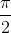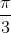Explanation:

For tangent and cotangent the period is given by the formula: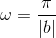wherecomes from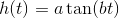.

Thus we see from our equation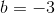and so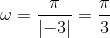.

### Example Question #33 : Trigonometry

What is the period of the trigonometric function given by: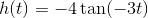?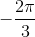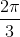Explanation:

To find the period of a tangent funciton use the following formula:wherecomes from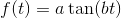.

thus we get thatso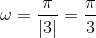### Example Question #34 : Trigonometry

What is the period of the following trigonometric function: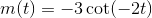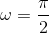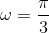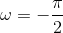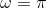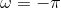Explanation:

To find the period of a tangent or cotangent function use the following formula:from the general tirogonometric formula: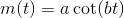Since we have,we have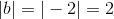.

Thus we get that